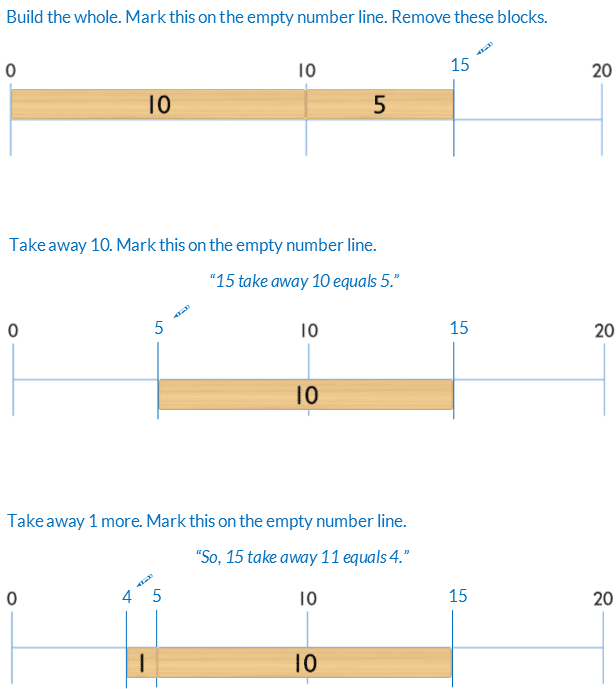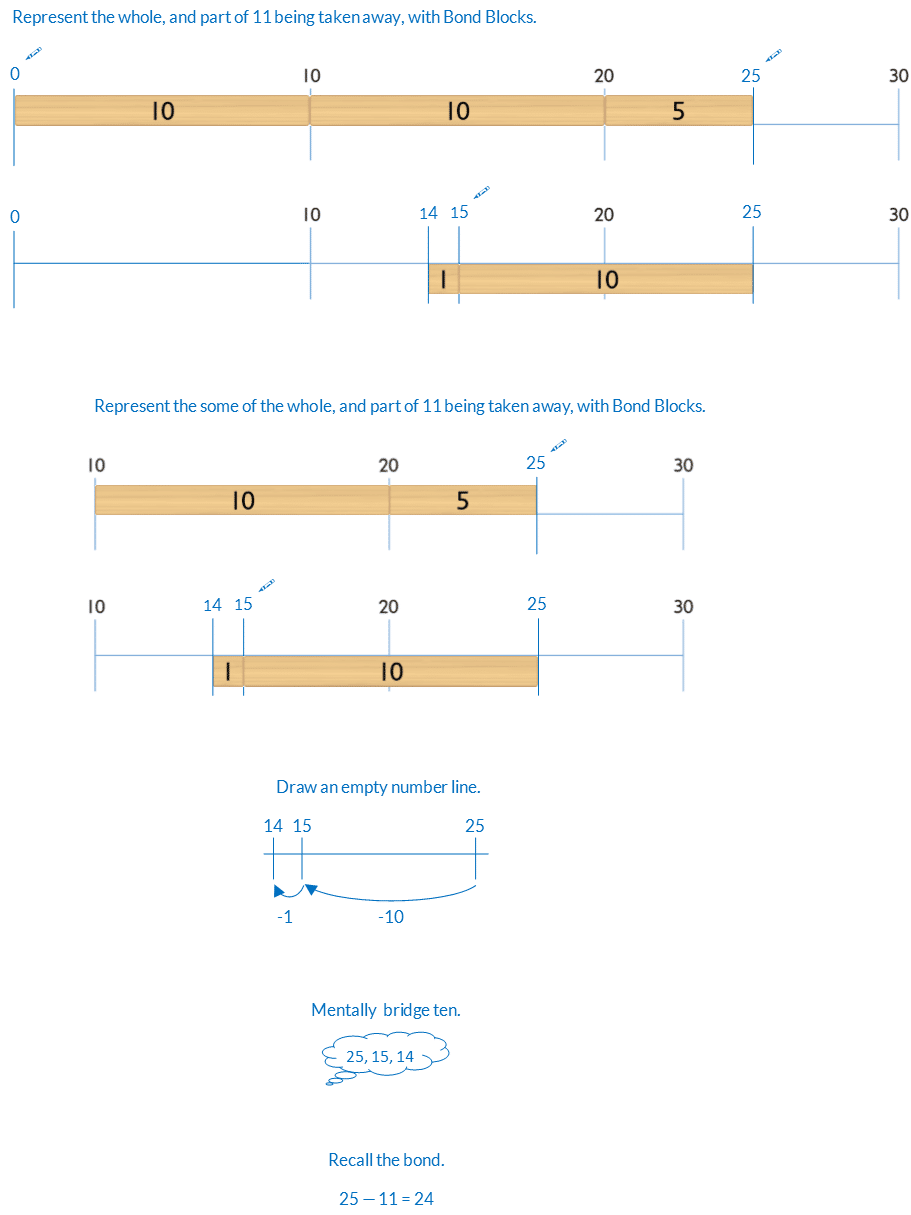## 70) Near Ten

Ten Plus Bonds: Strategy -11### Mathematics

To develop the strategy of taking away 11, by taking away 10, then another one, for wholes less than 20.

For example, to solve 15 – 11Prior knowledge of ten plus bonds, adding and subtracting ten and one, and subtraction solved using take away on an empty number line is needed.

### Language

• take away
• left
• equals## Differentiation

### A little easier

##### Counting order

Before using the Bonds of 11 to 20: Near Ten Strategy -11 as a game for pairs, students can use this as an activity board to individually calculate each question in counting order. Work clockwise around the spinner, from the 12 o’clock position. Do not flick the spinner. Assist students to identify patterns.

### A little harder

##### Near ten strategy -11

Play Bonds of 11 to 20: Near Ten Strategy -11 a little harder. In the game students have to subtract 11 from wholes in the twenties using the same strategy.##### Reduce scaffolds

The blocks are a scaffold to support calculation. If students can calculate without some or all of the blocks encourage this. Below is a progression of reducing scaffolding, from most to least support, using near ten for 25 – 11.### Progression

In the next activity students extend the near 10 strategy to subtracting a part of 9.

• This is more difficult than subtracting 11 because subtracting 11 involves only subtraction. The student subtracts 10, then subtracts one more. Their mental number line is continuing to take away moving left.
• Subtracting 9 is much more difficult because the student has to first take away ten, moving left on the mental number line (less), then add one, moving right (greater).

Go to

##### Activity 71

Ten Plus Bonds: Near Ten, Strategy -9